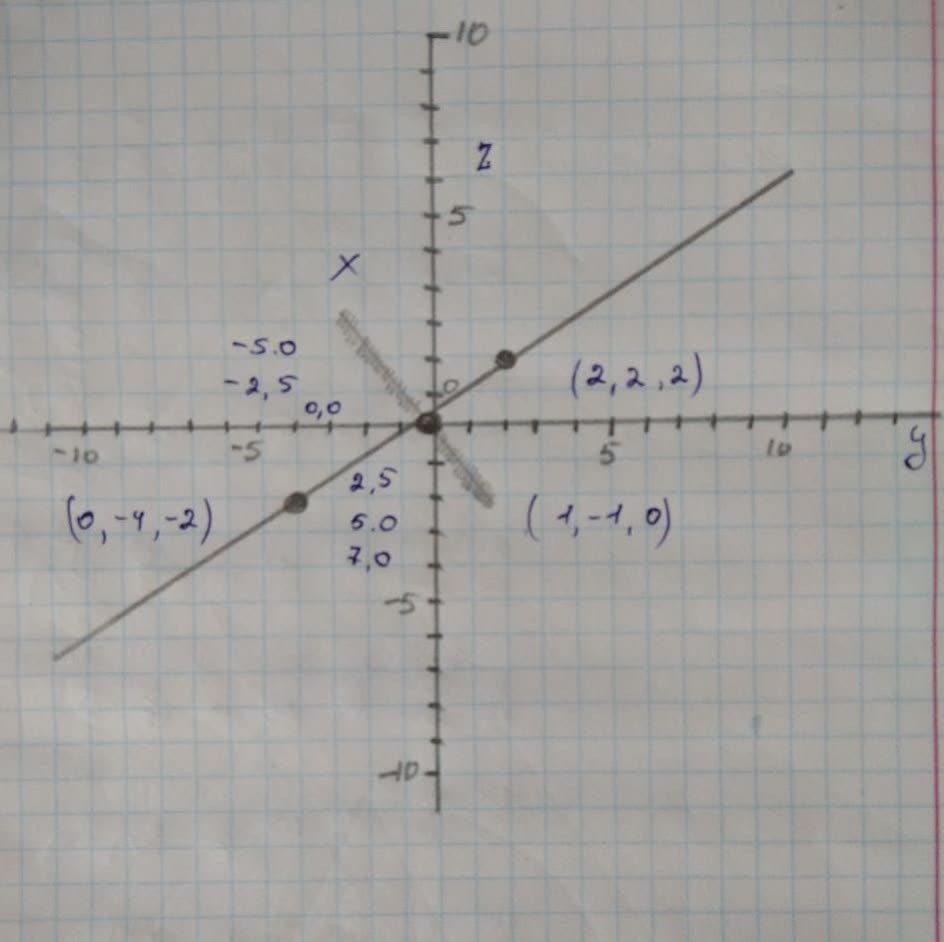Sketch the curve represented by the vector-valued function \r(t) =Antinazius 2021-11-25 Answered
Sketch the curve represented by the vector-valued function $$\displaystyle{r}{\left({t}\right)}={\left({t}+{1}\right)}{i}+{\left({3}{t}-{1}\right)}{j}+{2}{t}{k}$$ and give the orientation of the curve.

• Questions are typically answered in as fast as 30 minutes

Solve your problem for the price of one coffee

• Math expert for every subject
• Pay only if we can solve itKathleen Ashton
Step1
Given:
The vector-valued function $$\displaystyle{r}{\left({t}\right)}={\left({t}+{1}\right)}{i}+{\left({3}{t}-{1}\right)}{j}+{2}{t}{k}$$ and it gives the orientation of the curve.
Step2
The vector-valued function is given below as,
$$\displaystyle{r}{\left({t}\right)}={\left({t}+{1}\right)}{i}+{\left({3}{t}-{1}\right)}{j}+{2}{t}{k}$$
The first two parametric equations is given below as,
$$\displaystyle{x}={t}+{i}{\quad\text{and}\quad}{y}={3}{t}-{1}$$
The rectangular equation determines the two parametric equations.
The value of t from x=t+ 1 is calculated as below,
$$\displaystyle{x}={t}+{i}$$
$$\displaystyle{x}-{1}={t}+\cancel{{{1}}}-\cancel{{{1}}}$$
$$\displaystyle{t}={x}-{1}$$
The value of t=x-1.
Step3
On Substituting the value of t in y= 3t -1 to get
$$\displaystyle{y}={3}{\left({x}-{1}\right)}-{1}$$
$$\displaystyle={3}{x}-{3}-{1}$$
$$\displaystyle={3}{x}-{4}$$
Hence, the value of y=3x-4.
Thus, the rectangular equation is $$\displaystyle{y}={3}{x}-{4}$$
Hence, the curve is a straight line that is determined with respect to the space.
The curve move upwards as the value of t increases resulting in the third parametric equation that is z= 2t.
The vector valued function of the graph is being represented and the orientation is shown in the graph as,
$$\displaystyle{r}{\left({t}\right)}={\left({t}+{1}\right)}{i}+{\left({3}{t}-{1}\right)}{j}+{2}{t}{k}$$